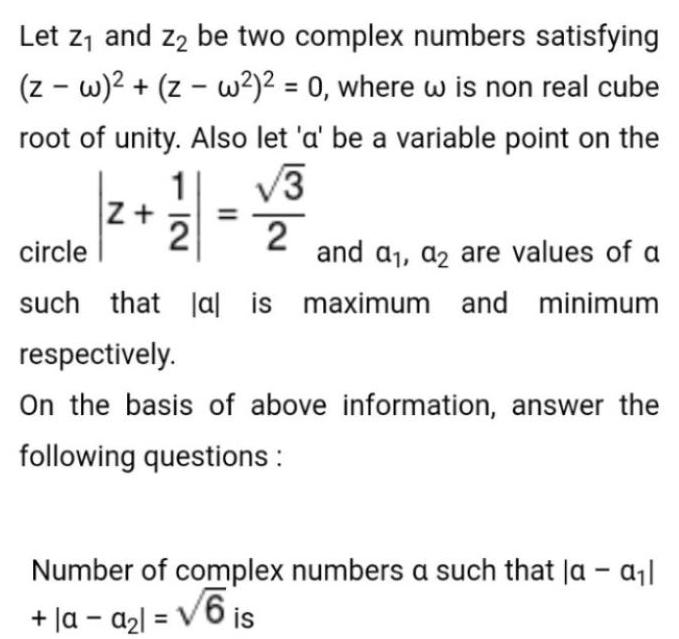Question:

# Let z₁ and z₂ be two complex numbers satisfying

Last updated: 7/29/2022Let z₁ and z₂ be two complex numbers satisfying (z-w)²+(z-w²)² = 0, where w is non real cube root of unity. Also let 'a' be a variable point on the circle |z+1/2|=√3/2 and a₁, a2 are values of a such that lal is maximum and minimum respectively. On the basis of above information, answer the following questions : Number of complex numbers a such that la - a₁l +|a -a₂l= √6 is